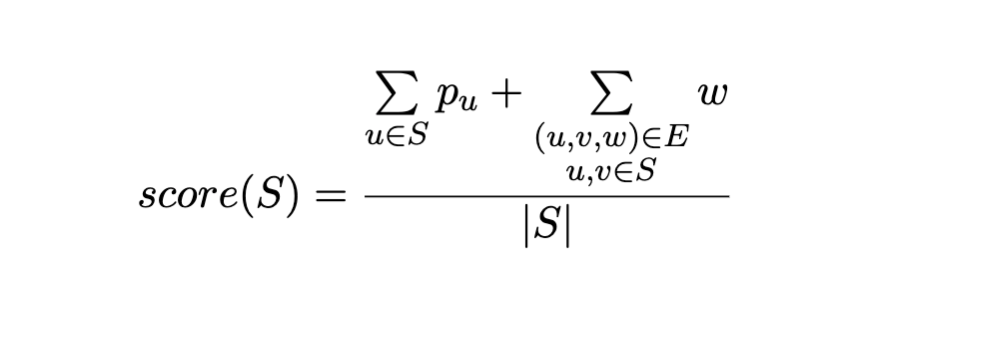### H - Heavy Graph

##### Time & Memory limits:(details)

You are given a weighted and undirected graph with $V$ vertices and $E$ edges. Each vertex also has an associated weight $p_1, p_2, \ldots , p_V$.

Find a subset $S$ of vertices with maximum score, where the score is defined as the sum of the weights of all nodes in $S$ and the weights of all the edges between nodes in $S$, divided by the number of vertices in $S$:#### Input

The first line contains two integers $V$ ($1 \le V \le 100$) and $E$ ($1 \le E \le 1000$).

The next line consist of $V$ integers $p_1, p_2, \ldots , p_V$ ($0 \le p_i \le 1000$).

Then $E$ lines, each containing three integers $u_i, v_i, w_i$ ($1 \le u_i \ne v_i \le V$, $1 \le w_i \le 1000$, $1 \le i \le E$) denoting an edge between nodes $u_i$ and $v_i$ with weight $w_i$.

There will be at most one undirected edge between any pair of vertices.

#### Output

Print a subset of vertices $S$ with maximum score as follows:

A line with an integer $| S |$, the number of vertices in $S$.

A line with $| S |$ integers separated by spaces: the vertices of $S$ in any order.

If there's more than one subgraph with the maximum score, print any of them.

#### Sample test(s)

Input
3 3 10 5 8 1 2 10 1 3 1 2 3 2
Output
2 1 2